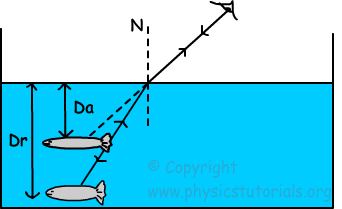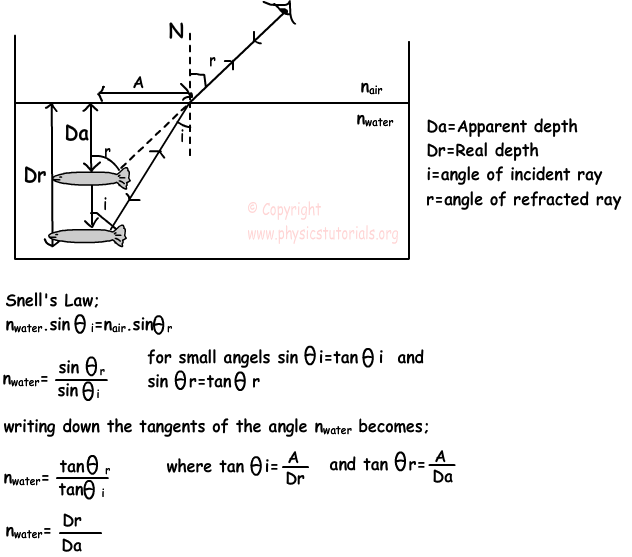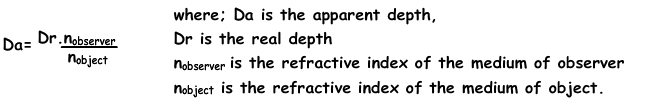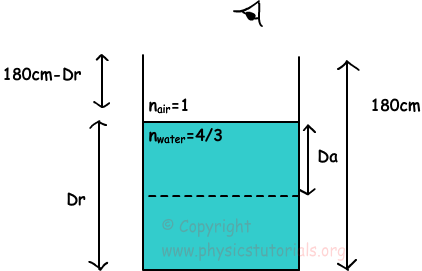## Apparent Depth Real Depth

Apparent Depth Real DepthPicture given above shows the difference between the real depth and apparent depth of the object under water. We see the objects closer than their real depth to the surface. We see objects only if the rays coming from them reaches our eyes. In this picture, ray coming from the fish reaches the observer’s eye after refraction. Thus, observer sees the image of the fish at the distance Da from the surface which is the apparent depth of the fish. On the contrary fish sees the objects away from their real distances. These are all results of the refraction of light. Following diagram helps us to calculate this apparent depth of the object under different mediums.As you can see refractive index of the water is equal to the ratio of real depth of fish to the apparent depth of it. More general form of our equation is given below;Example: An observer looks at the water tank and according to him half of the tank is filled with water. If the height of the tank is 180cm, find the real height of the water in the tank. (nwater=4/3)Da=Dr.nobserver/nobject

Da=3Dr/4

Observer sees the tank half filled thus;

3Dr/4=180-Dr

Dr=720/7=102,8cm and Da=3Dr/4=77,1cm

Optics Exams and Solutions

Author: